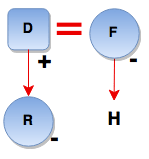# Blood Relations and Family Tree - Logical Reasoning (MCQ) Questions for Q. 29744

1. M \$ N means M is the mother of N
2. M # N means M is the father of N
3. M @ N means M is the husband of N
4. M % N means M is the daughter of N

Which of the following expressions indicate ‘R is the sister of H’?

- Published on 03 May 17

a. H \$ D @ F # R
b. R % D @ F \$ H
c. R \$ D @ F # H
d. None of these

ANSWER: R % D @ F \$ H

R % D @ F \$ H## ➨ Post your comment / Share knowledgeEnter the code shown above:

(Note: If you cannot read the numbers in the above image, reload the page to generate a new one.)